CLAT  >  Introduction to Electrochemistry & Electrolytic Cells

# Introduction to Electrochemistry & Electrolytic Cells - Notes | Study Current Affairs & General Knowledge - CLAT

 Table of contentsElectrochemistryMetallic & Electrolytic ConductorsElectrochemical CellsElectrolytic Cells1 Crore+ students have signed up on EduRev. Have you?

Electrochemistry

Electrochemistry is the subdiscipline of chemistry that deals with the study of the relationship between electrical energy and chemical changes.

Chemical reactions that involve the input or generation of electric currents are called electrochemical reactions. Such reactions are broadly classified into two categories:

1. Production of chemical change by electrical energy i.e. the phenomenon of electrolysis
2. Conversion of chemical energy into electrical energy. i.e., the generation of electricity by spontaneous redox reactions.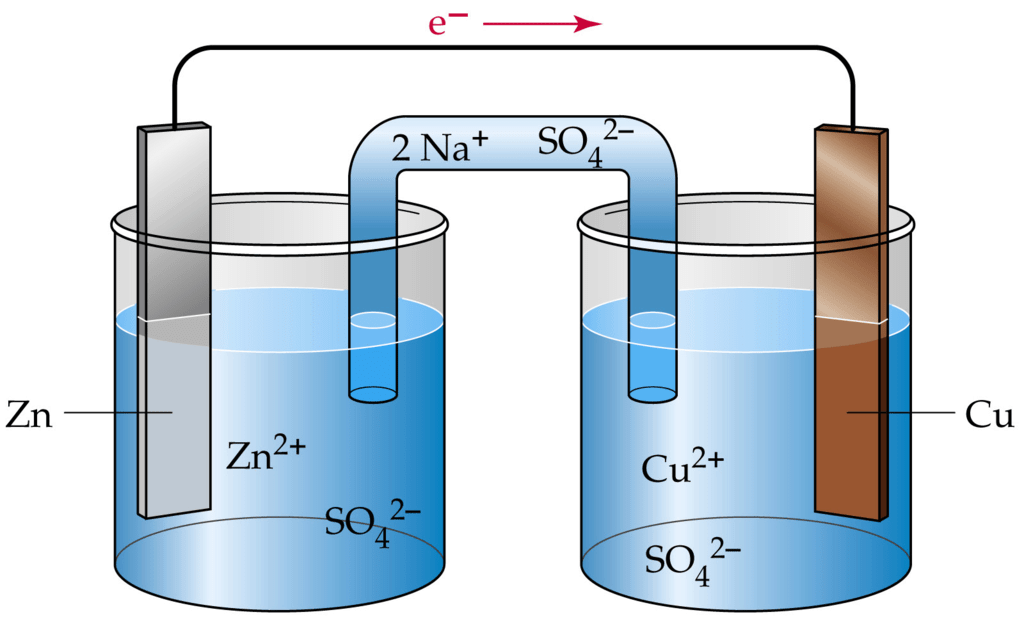An Electrochemical Cell

• Electricity can be produced when electrons move from one element to another in certain types of reactions (such as redox reactions).
• Typically, electrochemistry deals with the overall reactions when multiple redox reactions occur simultaneously, connected via some external electric current and a suitable electrolyte.
• In other words, electrochemistry is also concerned with chemical phenomena that involve charge separation (as seen commonly in liquids such as solutions). The dissociation of charge often involves charge transfer that occurs homogeneously or heterogeneously between different chemical species.

Metallic & Electrolytic Conductors

Solid and liquid substances, which are able to conduct the electric current, can be roughly divided into two categories i.e. metallic conductors and electrolytic conductors.

• Metallic Conductors
In metallic conductors or electronic conductors, the electric current is carried by the mobile electrons. When an electric potential is applied to metallic conductors, the electrons start moving in one direction while the positive ions remain stationary. Thus the flow of electricity is not accompanied by any appreciable movement of the matter.
• Electrolytic conductors
Electrolytic conductors or electrolytes are distinguished from metallic conductors by the fact that the current is carried by ions and not by electrons. The application of an electrical potential causes these charged particles of matter to move, the positive ions move towards the cathode and the negative ions move towards the anode.
Thus, the passage of an electric current through an electrolyte is always accompanied by a transfer of matter. This transfer is manifested by changes in concentration, and also by visible separation of material at the points where the electric current enters and leaves the electrolyte. Electrolytes generally employed are salts in molten form or salts dissolved in water.

The Distinction between Metallic and Electrolytic conduction.

 S.No. Metallic conduction Electrolytic conduction 1 Electric current flows by movement of electrons. Electric current flows by  movement of ions. 2 No chemical change Ions are oxidized or reduced at the electrodes. 3 It does not involve the transfer of any matter It involves the transfer of matter in the form of ions. 4 Ohm's law is followed Ohm's law is followed 5 Resistance increase with increase of temperature Resistance decreases with increase of temperature 6 Faraday law is not followed Faraday law is followed

Electrochemical Cells

In electrochemistry, spontaneous reaction (redox reaction) results in the conversion of chemical energy into electrical energy. The reverse process is also possible where a non-spontaneous chemical reaction occurs by supplying electricity. These interconversions are carried out in equipment called electrochemical cell.

• An electrochemical cell consists of two electrodes (metallic conductors) in contact with an electrolyte (an ionic conductor).
• An electrode and its electrolyte comprise an Electrode Compartment.

Types of Electrochemical Cell

Electrochemical Cells can be classified as:

(i) Electrolytic Cells: in which a non-spontaneous reaction is driven by an external source of current.
(ii) Galvanic Cells: which produce electricity as a result of a spontaneous cell reaction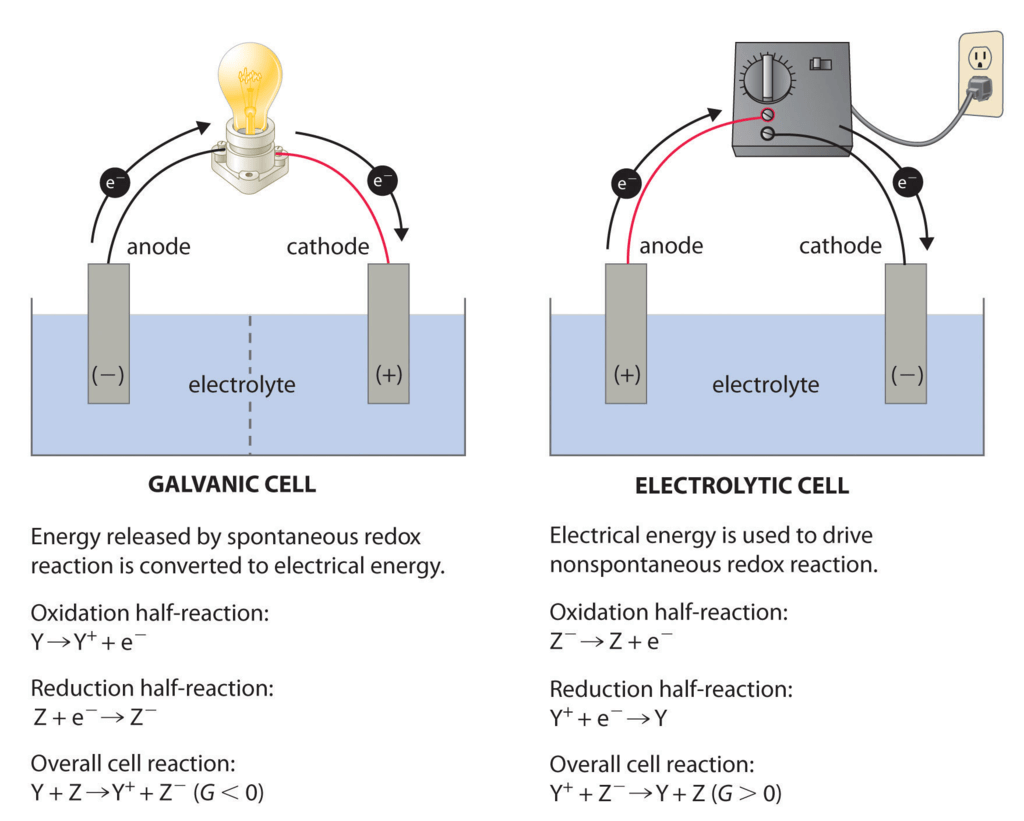Electrolytic Cells

The electrolytic cell converts electrical energy to chemical energy. Here the electrodes are dipped in an electrolytic solution containing cations and anions.

On supplying current the ions move towards electrodes of opposite polarity and simultaneous reduction and oxidation take place.

For example, in the electrolysis of molten sodium chloride, sodium chloride is melted (above 801oC), two electrodes are inserted into the melt, and an electric current is passed through the molten salt. The chemical reaction that takes place at the electrodes are:

● Sodium-ion migrates to the cathode, where sodium ion gains one electron and reduces to sodium metal.

Na+ + e→ Na

● Chloride ions migrate towards the anode where it loses one electron and gets oxidized to chlorine gas.

Cl→1/2 Cl2 + e

The overall reaction is the breakdown of sodium chloride into its elements

2NaCl→ 2Na(s) + Cl2(g)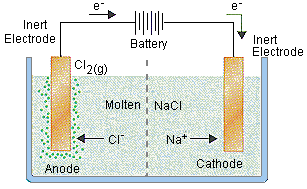Preferential Discharge of Ions

• When there are more than one cation or anion the process of discharge becomes competitive in nature. For example, in the electrolysis of NaCl solution, apart from Na+ and Clions the solution of sodium chloride also contains H+ and OH ions due to ionization of water.
• When the potential difference is applied between the two electrodes, Na+ and H+ ions move towards the cathode, and Cl and OH ions move towards the anode. At cathode Hions get reduce in preference to giving hydrogen gas because hydrogen has a higher reduction potential than sodium.
• Similarly, at the anode, Cl ions are oxidised in preference to OH– to give chlorine gas.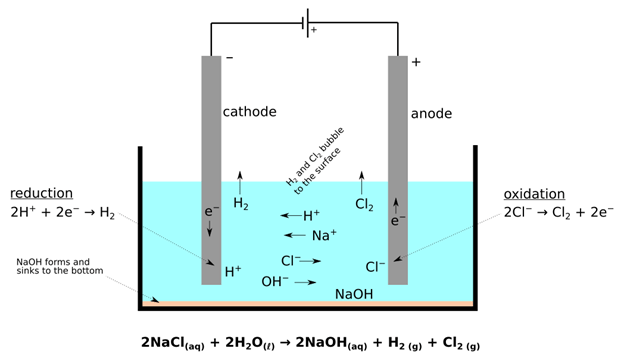Electrolysis

Electrolysis is a process of chemical decomposition of the electrolyte by the passage of electric current.

• It is carried out in a cell called electrolytic cell.
• The electrode at which oxidation takes place is called anode and the electrode at which reduction takes place is called cathode.
• The electrodes are named anode or cathode depending upon the reaction occurring on them and not on the basis of the sign of electrodes.
• Note: In a galvanic cell, the cathode is positive with respect to anode.
In an electrolytic cell, anode is made positive with respect to cathode.

Electrodes

 Anode Positive Loss of electronor oxidationtakes place Positivecurrententers Cathode Negative Gain of electronor reductiontakes place Currentleaves

Faraday established the relationship between the quantity of electricity passed through an electrolyte and the amount of material liberated or deposited at the electrode.

• The quantity of electricity is equal to the product the current strength and the time for which it is passed.
• The results of Faraday’s finding can be put in the form of two laws of electrolysis.

(i) First Law of Electrolysis

When an electric current is passed through an electrolyte, the amount of substance deposited is proportional to the quantity of electricity passed through the electrolyte.

• If W is the mass of the substance deposited by passing Q coulomb of charge, then according to the law, we have the relation:  W ∝ Q Remember that Q is not the amount of charge passed but it is the amount of charge utilized.
• A coulomb is the quantity of charge when a current of one ampere is passed for one second.  Thus, the amount of charge in coulombs,
Q = Current in amperes × time in seconds
= I × t
So W ∝ I × t
or W = Z × I × t
where Z is a constant, known as electro-chemical equivalent, and is characteristics of the substance deposited.
When a current of one ampere is passed for one second, i.e., one coulomb (Q = 1), then
W = Z
Thus, the electrochemical equivalent can be defined as the mass of the substance deposited by one coulomb of charge or by one ampere of current passed for one second.

(ii) Second Law of Electrolysis

The amount of different substances deposited or dissolved by the same quantity of electricity are proportional to their respective chemical equivalent weights.

• W = Z × Q
Thus, E = Z × 96500
or Z =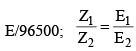Charge and Potential Difference

As one g-equivalent of an ion is liberated by 96500 coulombs, it follows that the charge carried by one g-equivalent of an ion is 96500 coulomb.  If the valency of an ion is ‘n’, the one mole of these ions will carry a charge of nF coulomb.  One g-mole of an ion contains 6.02 × 1023 ions.
Then, the charge carried by anion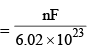coulomb

For n = 1,  The fundamental unit of charge =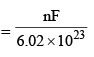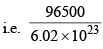= 1.6 × 10–19 coulomb

or 1 coulomb* = 6.25 × 1018 electrons

The rate of flow of electric charge through a conductor is called the electric current.
1 coulomb = 1 ampere-second
Electric current =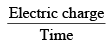1 ampere =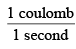Volt is a unit of electrical potential difference, it is defined as potential energy per unit charge.
1 volt =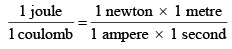Electrical energy = potential difference × Quantity of charge
= V × Q
= V × I × t (I = ampere; t = second)
= Watt-second

One faraday is the charge required to liberate or deposit one gm equivalent of a substance at the corresponding electrode.
Faraday’s Law for Gaseous Electrolytic Product We know W = ZQ = Z I t
W =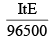..(i)
where Z = E/96500

Equation (i) is used to calculate the mass of a solid substance dissolved or deposited at an electrode.
For the gases, we use

V =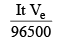..(ii)
where V = volume of gas evolved at S.T.P. at an electrode
Ve =  Equivalent volume
=  Volume of gas evolved at an electrode at S.T.P. by 1 Faraday charge.

The document Introduction to Electrochemistry & Electrolytic Cells - Notes | Study Current Affairs & General Knowledge - CLAT is a part of the CLAT Course Current Affairs & General Knowledge.
All you need of CLAT at this link: CLAT

## Current Affairs & General Knowledge

135 videos|627 docs|35 tests
 Use Code STAYHOME200 and get INR 200 additional OFF

## Current Affairs & General Knowledge

135 videos|627 docs|35 tests

Track your progress, build streaks, highlight & save important lessons and more!

,

,

,

,

,

,

,

,

,

,

,

,

,

,

,

,

,

,

,

,

,

;